# Fixed-Displacement Motor

(To be removed) Hydraulic-to-mechanical power conversion device

The Hydraulics (Isothermal) library will be removed in a future release. Use the Isothermal Liquid library instead. (since R2020a)

•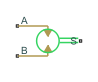Libraries:
Simscape / Fluids / Hydraulics (Isothermal) / Pumps and Motors

## Description

The Fixed-Displacement Motor block represents a device that extracts power from a hydraulic (isothermal liquid) network and delivers it to a mechanical rotational network. The motor displacement is fixed at a constant value that you specify through the Displacement parameter.

Ports A and B represent the motor inlet and outlet, respectively. Port S represents the motor drive shaft. During normal operation, the angular velocity at port S is positive if the pressure drop from port A to port B is positive also. This operation mode is referred to here as forward motor.

Operation Modes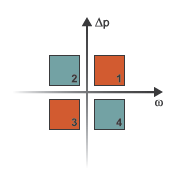A total of four operation modes are possible. The working mode depends on the pressure drop from port A to port B (Δp) and on the angular velocity at port S (ω). The Operation Modes figure maps the modes to the octants of a Δp-ω-D chart. The modes are labeled 1–4:

• Mode 1: forward motor — A positive pressure drop generates a positive shaft angular velocity.

• Mode 2: reverse pump — A negative shaft angular velocity generates a negative pressure gain (shown in the figure as a positive pressure drop).

• Mode 3: reverse motor — A negative pressure drop generates a negative shaft angular velocity.

• Mode 4: forward pump — A positive shaft angular velocity generates a positive pressure gain (shown in the figure as a negative pressure drop).

The response time of the motor is considered negligible in comparison with the system response time. The motor is assumed to reach steady state nearly instantaneously and is treated as a quasi-steady component.

### Block Variants and Loss Parameterizations

The motor model accounts for power losses due to leakage and friction. Leakage is internal and occurs between the motor inlet and outlet only. The block computes the leakage flow rate and friction torque using your choice of five loss parameterizations. You select a parameterization using block variants and, in the `Analytical or tabulated data` case, the Leakage and friction parameterization parameter.

Loss ParameterizationsThe block provides three Simscape™ variants to select from. To change the active block variant, use the parameter. The available variants are:

• `Analytical or tabulated data` — Obtain the mechanical and volumetric efficiencies or losses from analytical models based on nominal parameters or from tabulated data. Use the Leakage and friction parameterization parameter to select the exact input type.

• `Input efficiencies` — Provide the mechanical and volumetric efficiencies directly through physical signal input ports.

• `Input losses` — Provide the mechanical and volumetric losses directly through physical signal input ports. The mechanical loss is defined as the internal friction torque. The volumetric loss is defined as the internal leakage flow rate.

### Flow Rate and Driving Torque

The volumetric flow rate required to power the motor is

`$q={q}_{\text{Ideal}}+{q}_{\text{Leak}},$`

where:

• q is the net volumetric flow rate.

• qIdeal is the ideal volumetric flow rate.

• qLeak is the internal leakage volumetric flow rate.

The torque generated at the motor is

`$\tau ={\tau }_{\text{Ideal}}-{\tau }_{\text{Friction}},$`

where:

• τ is the net torque.

• τIdeal is the ideal torque.

• τFriction is the friction torque.

Ideal Flow Rate and Ideal Torque

The ideal volumetric flow rate is

`${q}_{\text{Ideal}}=D\omega ,$`

and the ideal generated torque is

`${\tau }_{\text{Ideal}}=D\Delta p,$`

where:

• D is the specified value of the Displacement block parameter.

• ω is the instantaneous angular velocity of the rotary shaft.

• Δp is the instantaneous pressure drop from inlet to outlet.

Leakage Flow Rate and Friction Torque

The internal leakage flow rate and friction torque calculations depend on the block variant selected. If the block variant is ```Analytical or tabulated data```, the calculations depend also on the Leakage and friction parameterization parameter setting. There are five possible permutations of block variant and parameterization settings.

Case 1: Analytical Efficiency Calculation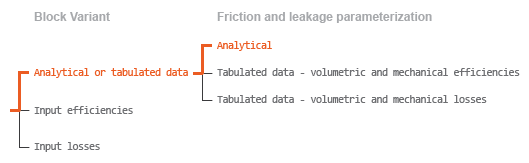If the active block variant is ```Analytical or tabulated data``` and the Leakage and friction parameterization parameter is set to `Analytical`, the leakage flow rate is

`${q}_{\text{Leak}}={K}_{\text{HP}}\Delta p,$`

and the friction torque is

`${\tau }_{\text{Friction}}=\left({\tau }_{0}+K{\text{​}}_{\text{TP}}|\Delta p|\right)\mathrm{tanh}\left(\frac{4\omega }{{\omega }_{\text{Threshold}}}\right),$`

where:

• KHP is the Hagen-Poiseuille coefficient for laminar pipe flows. This coefficient is computed from the specified nominal parameters.

• KTP is the specified value of the Friction torque vs pressure drop coefficient block parameter.

• τ0 is the specified value of the No-load torque block parameter.

• ωThreshold is the threshold angular velocity for the motor-pump transition. The threshold angular velocity is an internally set fraction of the specified value of the Nominal shaft angular velocity block parameter.

The Hagen-Poiseuille coefficient is determined from nominal fluid and component parameters through the equation

`${K}_{\text{HP}}=\frac{{\nu }_{\text{Nom}}}{\rho v}\frac{\text{\hspace{0.17em}}{\rho }_{\text{Nom}}{\omega }_{\text{Nom}}{D}_{\text{Max}}}{\Delta {p}_{\text{Nom}}}\left(\frac{1}{{\eta }_{\text{v,Nom}}}-1\right),$`

where:

• νNom is the specified value of the Nominal kinematic viscosity block parameter. This is the kinematic viscosity at which the nominal volumetric efficiency is specified.

• ρNom is the specified value of the Nominal fluid density block parameter. This is the density at which the nominal volumetric efficiency is specified.

• ωNom is the specified value of the Nominal shaft angular velocity block parameter. This is the angular velocity at which the nominal volumetric efficiency is specified.

• ρ is the actual fluid density in the attached hydraulic (isothermal liquid) network. This density can differ from the specified value of the Nominal fluid density block parameter.

• v is the kinematic viscosity of the fluid associated with the fluid network.

• ΔpNom is the specified value of the Nominal pressure drop block parameter. This is the pressure drop at which the nominal volumetric efficiency is specified.

• ηv,Nom is the specified value of the Volumetric efficiency at nominal conditions block parameter. This is the volumetric efficiency corresponding to the specified nominal conditions.

Case 2: Efficiency Tabulated Data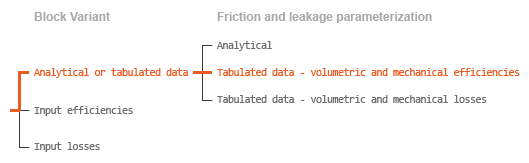If the active block variant is ```Analytical or tabulated data``` and the Leakage and friction parameterization parameter is set to ```Tabulated data — volumetric and mechanical efficiencies```, the leakage flow rate is

`${q}_{\text{Leak}}={q}_{\text{Leak,Motor}}\frac{\left(1+\alpha \right)}{2}+{q}_{\text{Leak,Pump}}\frac{\left(1-\alpha \right)}{2},$`

and the friction torque is

`${\tau }_{\text{Friction}}={\tau }_{\text{Friction,Motor}}\frac{1+\alpha }{2}+{\tau }_{\text{Friction,Pump}}\frac{1-\alpha }{2},$`

where:

• α is a numerical smoothing parameter for the motor-pump transition.

• qLeak,Motor is the leakage flow rate in motor mode.

• qLeak,Pump is the leakage flow rate in pump mode.

• τFriction,Motor is the friction torque in motor mode.

• τFriction,Pump is the friction torque in pump mode.

The smoothing parameter α is given by the hyperbolic function

`$\alpha =\text{tanh}\left(\frac{4\Delta p}{\Delta {p}_{\text{Threshold}}}\right)·\text{tanh}\left(\frac{4\omega }{{\omega }_{\text{Threshold}}}\right),$`

where:

• ΔpThreshold is the specified value of the Pressure drop threshold for motor-pump transition block parameter.

• ωThreshold is the specified value of the Angular velocity threshold for motor-pump transition block parameter.

The leakage flow rate is computed from efficiency tabulated data through the equation

`${q}_{\text{Leak,Motor}}=\left(1-{\eta }_{\text{v}}\right)q,$`

in motor mode and through the equation

`${q}_{\text{Leak,Pump}}=-\left(1-{\eta }_{\text{v}}\right){q}_{\text{Ideal}}.$`

in pump mode, where:

• ηv is the volumetric efficiency obtained through interpolation or extrapolation of the Volumetric efficiency table, e_v(dp,w) parameter data.

Similarly, the friction torque is computed from efficiency tabulated data through the equation

`${\tau }_{\text{Friction,Motor}}=\left(1-{\eta }_{\text{m}}\right){\tau }_{\text{Ideal}},$`

in motor mode and through the equation

`${\tau }_{\text{Friction,Pump}}=-\left(1-{\eta }_{\text{m}}\right)\tau .$`

in pump mode, where:

• ηm is the mechanical efficiency obtained through interpolation or extrapolation of the Mechanical efficiency table, e_m(dp,w) parameter data.

Case 3: Loss Tabulated Data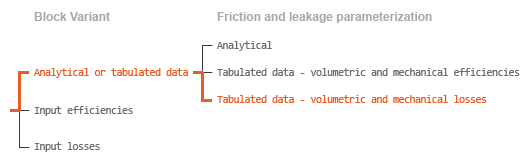If the active block variant is ```Analytical or tabulated data``` and the Leakage and friction parameterization parameter is set to ```Tabulated data — volumetric and mechanical losses```, the leakage flow rate equation is

`${q}_{\text{Leak}}={q}_{\text{Leak}}\left(\Delta p,\omega \right).$`

and the friction torque equation is

`${\tau }_{\text{Friction}}={\tau }_{\text{Friction}}\left(\Delta p,\omega \right),$`

where qLeak(Δp,ω) and τFriction(Δp,ω) are the volumetric and mechanical losses, obtained through interpolation or extrapolation of the Volumetric loss table, q_loss(dp,w) and Mechanical loss table, torque_loss (dp,w) parameter data.

Case 4: Efficiency Physical Signal InputsIf the active block variant is `Input efficiencies`, the leakage flow rate and friction torque calculations are as described for efficiency tabulated data (case 2). The volumetric and mechanical efficiency lookup tables are replaced with physical signal inputs that you specify through ports EV and EM.

Case 5: Loss Physical Signal InputsIf the block variant is `Input losses`, the leakage flow rate and friction torque calculations are as described for loss tabulated data (case 3). The volumetric and mechanical loss lookup tables are replaced with physical signal inputs that you specify through ports LV and LM.

### Characteristic Curve Visualization

If the block variant is set to ```Analytical or tabulated data```, you can plot a variety of performance, efficiency, and loss curves from simulation data and component parameters. Use the context-sensitive menu of the block to plot the characteristic curves. Right-click the block to open the menu and select Fluids > Plot characteristic. A test harness opens with instructions on how to generate the curves.

### Assumptions

• Fluid compressibility is negligible.

• Loading on the motor shaft due to inertia, friction, and spring forces is negligible.

## Ports

### Input

expand all

Physical signal input port for the volumetric efficiency coefficient. The input signal has an upper bound at the Maximum volumetric efficiency parameter value and a lower bound at the Minimum volumetric efficiency parameter value.

#### Dependencies

This port is exposed only when the block variant is set to `Input efficiencies`.

Physical signal input port for the mechanical efficiency coefficient. The input signal has an upper bound at the Maximum mechanical efficiency parameter value and a lower bound at the Minimum mechanical efficiency parameter value.

#### Dependencies

This port is exposed only when the block variant is set to `Input efficiencies`.

Physical signal input port for the volumetric loss, defined as the internal leakage flow rate between the motor inlets.

#### Dependencies

This port is exposed only when the block variant is set to `Input losses`.

Physical signal input port for the mechanical loss, defined as the friction torque on the rotating motor shaft.

#### Dependencies

This port is exposed only when the block variant is set to `Input losses`.

### Conserving

expand all

Hydraulic (isothermal liquid) port representing the motor inlet.

Hydraulic (isothermal liquid) port representing the motor outlet.

Mechanical rotational port representing the motor shaft.

## Parameters

expand all

The exposed block parameters depend on the active block variant. See Block Variants and Loss Parameterizations.

Set the variant for mechanical and volumetric efficiencies or losses for the block.

Variant 1: `Analytical or tabulated data`

Fluid volume displaced per unit shaft rotation angle. The displacement is fixed at this value during simulation. The specified value must be greater than zero.

Parameterization used to compute flow-rate and torque losses due to internal leaks and friction. The `Analytical` parameterization relies on nominal parameters generally available from component data sheets. The remaining, tabular, options rely on lookup tables to map pressure drop and angular velocity to component efficiencies or losses. The tabular options include:

• ```Tabulated data — volumetric and mechanical efficiencies```

• ```Tabulated data — volumetric and mechanical losses```

Angular velocity of the rotating shaft at which the component’s nominal volumetric efficiency is known. Nominal parameters are typically published for standard operating conditions in manufacturer’s data sheets. The block uses this parameter to calculate, using simple linear functions, the leakage flow rate and friction torque.

#### Dependencies

This parameter is enabled when the Leakage and friction parameterization parameter is set to `Analytical`.

Pressure drop from inlet to outlet at which the component’s nominal volumetric efficiency is known. Nominal parameters are typically published for standard operating conditions in manufacturer’s data sheets. The block uses this parameter to calculate, using a simple linear function, the internal leakage flow rate.

#### Dependencies

This parameter is enabled when the Leakage and friction parameterization parameter is set to `Analytical`.

Kinematic viscosity of the hydraulic fluid at which the component’s nominal volumetric efficiency is known. Nominal parameters are typically published for standard operating conditions in manufacturer’s data sheets. The block uses this parameter to calculate, using a simple linear function, the internal leakage flow rate.

#### Dependencies

This parameter is enabled when the Leakage and friction parameterization parameter is set to `Analytical`.

Mass density of the hydraulic fluid at which the component’s nominal volumetric efficiency is known. Nominal parameters are typically published for standard operating conditions in manufacturer’s data sheets. The block uses this parameter to calculate, using a simple linear function, the internal leakage flow rate.

#### Dependencies

This parameter is enabled when the Leakage and friction parameterization parameter is set to `Analytical`.

Volumetric efficiency, defined as the ratio of actual to ideal volumetric flow rates, at the specified nominal conditions. Nominal parameters are typically published for standard operating conditions in manufacturer’s data sheets. The block uses this parameter to calculate, using a simple linear function, the internal leakage flow rate.

#### Dependencies

This parameter is enabled when the Leakage and friction parameterization parameter is set to `Analytical`.

Torque required to overcome seal friction and induce rotation of the mechanical shaft. This torque is the load-independent component of the total friction torque.

#### Dependencies

This parameter is enabled when the Leakage and friction parameterization parameter is set to `Analytical`.

Proportionality constant between the friction torque on the mechanical shaft and the pressure drop from inlet to outlet.

#### Dependencies

This parameter is enabled when the Leakage and friction parameterization parameter is set to ```Tabulated data — volumetric and mechanical efficiencies```.

Simulation warning mode for invalid pressures at the component ports. Select `Warning` to be notified when pressure falls below a minimum specified value. The warning can be useful in models where pressure can fall below the saturated vapor pressure of the hydraulic fluid, causing cavitation to occur.

Lower bound of the pressure validity range. A warning is issued if pressure falls below the specified value.

#### Dependencies

This parameter is enabled when the parameter is set to `Warning`.

M-element vector of pressure gains at which to specify the efficiency tabular data. The vector size, M, must be two or greater. The vector elements need not be uniformly spaced. However, they must monotonically increase in value from left to right.

#### Dependencies

This parameter is enabled when the Leakage and friction parameterization parameter is set to ```Tabulated data — volumetric and mechanical efficiencies```.

N-element vector of shaft angular velocities at which to specify the efficiency tabular data. The vector size, N, must be two or greater. The vector elements need not be uniformly spaced. However, they must monotonically increase in value from left to right.

#### Dependencies

This parameter is enabled when the Leakage and friction parameterization parameter is set to ```Tabulated data — volumetric and mechanical efficiencies```.

M-by-N matrix with the volumetric efficiencies at the specified fluid pressure drops and shaft angular velocities. The efficiencies must fall in the range of 0–1. M and N are the sizes of the specified lookup-table vectors:

• M is the number of vector elements in the Pressure drop vector for efficiencies, dp parameter.

• N is the number of vector elements in the parameter.

#### Dependencies

This parameter is enabled when the Leakage and friction parameterization parameter is set to ```Tabulated data — volumetric and mechanical efficiencies```.

M-by-N matrix with the mechanical efficiencies corresponding to the specified fluid pressure drops and shaft angular velocities. The efficiencies must fall in the range of 0–1. M and N are the sizes of the specified lookup-table vectors:

• M is the number of vector elements in the Pressure drop vector for efficiencies, dp parameter.

• N is the number of vector elements in the parameter.

#### Dependencies

This parameter is enabled when the Leakage and friction parameterization parameter is set to ```Tabulated data — volumetric and mechanical efficiencies```.

Pressure drop from inlet to outlet below which the component begins to transition between motoring and pumping modes. A hyperbolic `Tanh` function transforms the leakage flow rate and friction torque such that the transition is continuous and smooth.

#### Dependencies

This parameter is enabled when the Leakage and friction parameterization parameter is set to ```Tabulated data — volumetric and mechanical efficiencies```.

Shaft angular velocity below which the component begins to transition between motoring and pumping modes. A hyperbolic `Tanh` function transforms the leakage flow rate and friction torque such that the transition is continuous and smooth.

#### Dependencies

This parameter is enabled when the Leakage and friction parameterization parameter is set to ```Tabulated data — volumetric and mechanical efficiencies```.

Simulation warning mode for operating conditions outside the range of tabulated data. Select `Warning` to be notified when the fluid pressure gain or shaft angular velocity cross outside the specified tabular data. The warning does not cause simulation to stop.

#### Dependencies

This parameter is enabled when the Leakage and friction parameterization parameter is set to ```Tabulated data — volumetric and mechanical efficiencies``` or ```Tabulated data — volumetric and mechanical losses```.

M-element vector of pressure drops at which to specify the loss tabular data. The vector size, M, must be two or greater. The vector elements need not be uniformly spaced. However, they must monotonically increase in value from left to right.

#### Dependencies

This parameter is enabled when the Leakage and friction parameterization parameter is set to ```Tabulated data — volumetric and mechanical losses```.

N-element vector of shaft angular velocities at which to specify the component loss data. The vector size, N, must be two or greater. The vector elements need not be uniformly spaced. However, they must monotonically increase in value from left to right.

#### Dependencies

This parameter is enabled the Leakage and friction parameterization parameter is set to ```Tabulated data — volumetric and mechanical losses```.

M-by-N matrix with the volumetric gains at the specified fluid pressure drops and shaft angular velocities. Volumetric loss is defined here as the internal leakage volumetric flow rate between port A and port B. M and N are the sizes of the specified lookup-table vectors:

• M is the number of vector elements in the Pressure drop vector for losses, dp parameter.

• N is the number of vector elements in the parameter.

#### Dependencies

This parameter is enabled when the Leakage and friction parameterization parameter is set to ```Tabulated data — volumetric and mechanical losses```.

M-by-N matrix with the mechanical losses at the specified fluid pressure drops and shaft angular velocities. Mechanical loss is defined here as the friction torque due to seals and internal components. M and N are the sizes of the specified lookup-table vectors:

• M is the number of vector elements in the Pressure drop vector for losses, dp parameter.

• N is the number of vector elements in the parameter.

#### Dependencies

This parameter is enabled when the Leakage and friction parameterization parameter is set to ```Tabulated data — volumetric and mechanical losses```.

Variant 2: `Input efficiencies`

Fluid volume displaced per unit shaft rotation angle. The displacement is fixed at this value during simulation. The specified value must be greater than zero.

Smallest allowed value of the volumetric efficiency. The input from physical signal port EV saturates at the specified value. If the input signal falls below the minimum volumetric efficiency, the volumetric efficiency is set to the minimum volumetric efficiency.

Largest allowed value of the volumetric efficiency. The input from physical signal port EV saturates at the specified value. If the input signal rises above the maximum volumetric efficiency, the volumetric efficiency is set to the maximum volumetric efficiency.

Smallest allowed value of the mechanical efficiency. The input from physical signal port EM saturates at the specified value. If the input signal falls below the minimum mechanical efficiency, the mechanical efficiency is set to the minimum mechanical efficiency.

Largest allowed value of the mechanical efficiency. The input from physical signal port EM saturates at this value. If the input signal rises above the maximum mechanical efficiency, the mechanical efficiency is set to the maximum mechanical efficiency.

Pressure drop from inlet to outlet below which the component begins to transition between motoring and pumping modes. A hyperbolic `Tanh` function transforms the leakage flow rate and friction torque such that the transition is continuous and smooth.

Shaft angular velocity below which the component begins to transition between motoring and pumping modes. A hyperbolic `Tanh` function transforms the leakage flow rate and friction torque such that the transition is continuous and smooth.

Simulation warning mode for invalid pressures at the component ports. Select `Warning` to be notified when pressure falls below a minimum specified value. The warning can be useful in models where pressure can fall below the saturated vapor pressure of the hydraulic fluid, causing cavitation to occur.

Lower bound of the pressure validity range. A warning is issued if pressure falls below the specified value.

#### Dependencies

This parameter is enabled when the parameter is set to `Warning`.

Variant 3: Input losses

Fluid volume displaced per unit shaft rotation angle. The displacement is fixed at this value during simulation. The specified value must be greater than zero.

Pressure drop from inlet to outlet below which the component begins to transition between motoring and pumping modes. A hyperbolic `Tanh` function transforms the leakage flow rate and friction torque such that the transition is continuous and smooth.

Shaft angular velocity below which the component begins to transition between motoring and pumping modes. A hyperbolic `Tanh` function transforms the leakage flow rate and friction torque such that the transition is continuous and smooth.

Simulation warning mode for operating conditions outside the motoring mode. A warning is issued if the motor transitions to pumping mode. Select `Warning` to be notified when this transition occurs. The warning does not cause simulation to stop.

Simulation warning mode for invalid pressures at the component ports. Select `Warning` to be notified when pressure falls below a minimum specified value. The warning can be useful in models where pressure can fall below the saturated vapor pressure of the hydraulic fluid, causing cavitation to occur.

Lower bound of the pressure validity range. A warning is issued if pressure falls below the specified value.

#### Dependencies

This parameter is enabled when the parameter is set to `Warning`.

## Version History

Introduced in R2006b

collapse all

### R2023a: To be removed

The Hydraulics (Isothermal) library will be removed in a future release. Use the Isothermal Liquid library instead.